# Excel tutorial â€“ extract text or number by specific position

You may find that in many situations, all that is required of you is to extract the useful content from a sentence or a text string contained within a cell. For example, you may need to extract the province from the address, extract the email address from a sentence, or extract the courier bill number from a conversation. This article provides a collection of alternative ways that can assist in extracting text or numbers from a cell in Excel based on the precise position of the cell. The extraction is narrowed down to a certain place in the cell.

## Extract Text by position

This section compiles the typical locations within a cell from which text can be extracted and presents the relevant methods that can be used to manage those locations step by step.

## Extract first two characters from left

Step 1

If you have a list of text strings in column A, like the one shown in the screenshot below, you can use the following formulas to extract the first two characters from each string to create a new string.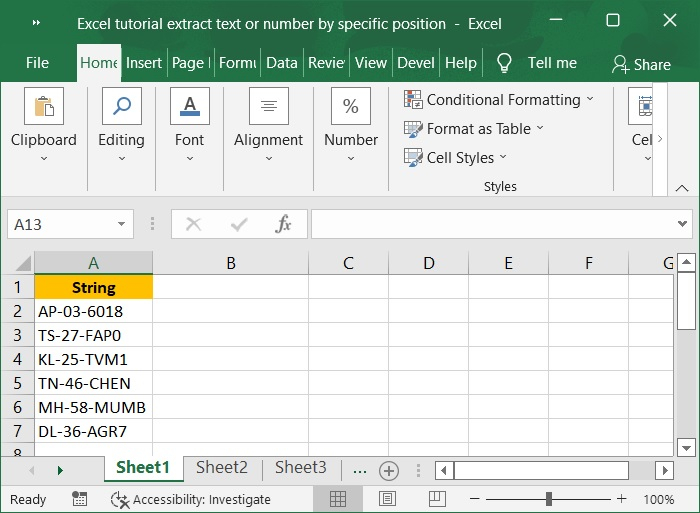Step 2

To extract the first two characters from the above string in Column A, we need to use LEFT () function.

For example, if we are trying to extract first two characters from the row A2, then write â€ś=LEFT(A2,2)â€ť in cell B2 and press enter. Refer to below screenshot for the same.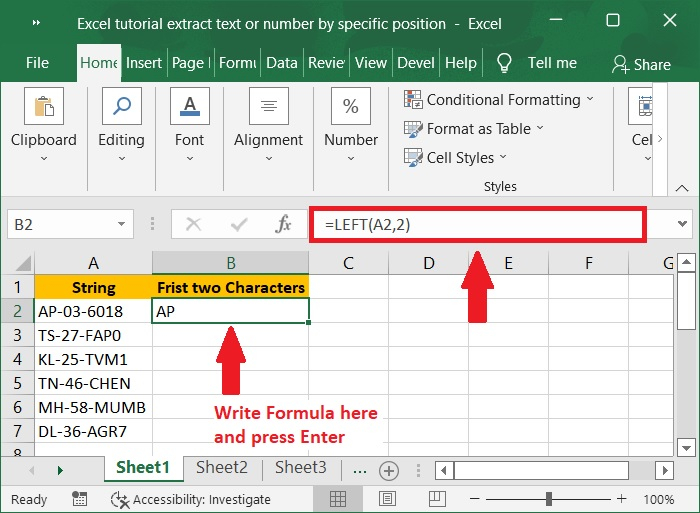Step 3

To apply the formula to the other cells, select the cell that contains the result, then drag the AutoFill Handle down. Below is the screenshot for the same.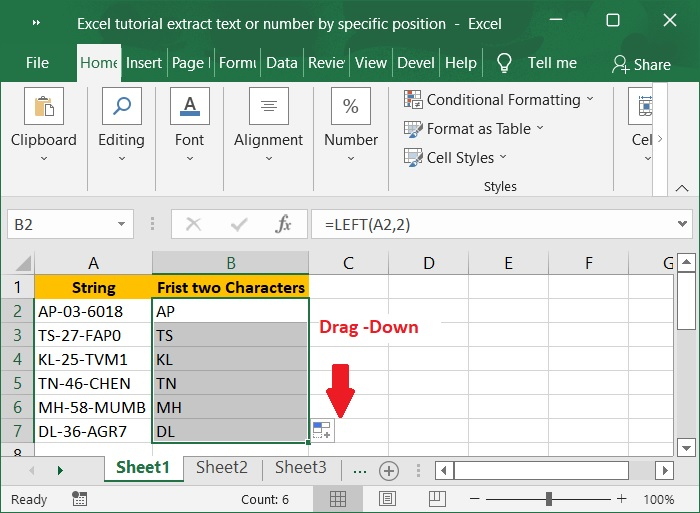## Extract Last four characters from right

Step 1

If you have a list of text strings in column A, like the one shown in the screenshot below, you can use the following formulas to extract the last four characters from each string to create a new string.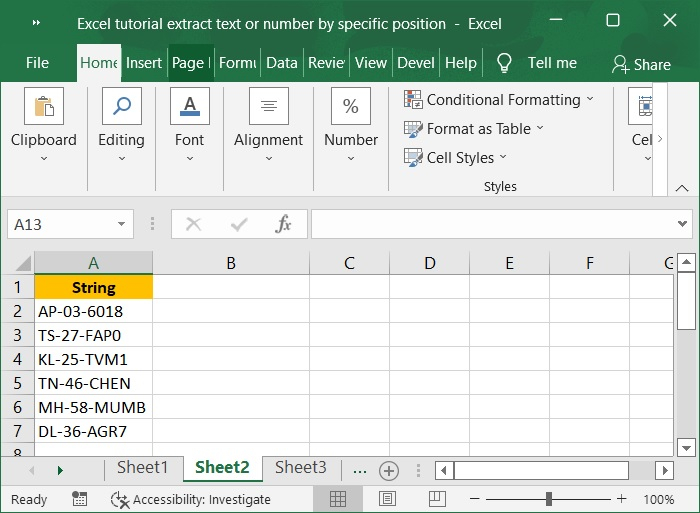Step 2

To extract the last four characters from the above string in Column A, we need to use RIGHT () function.

For example, if we are trying to extract last four characters from the row A2, then write â€ś=RIGHT(A2,4)â€ť in cell B2 and press enter. Refer to below screenshot for the same.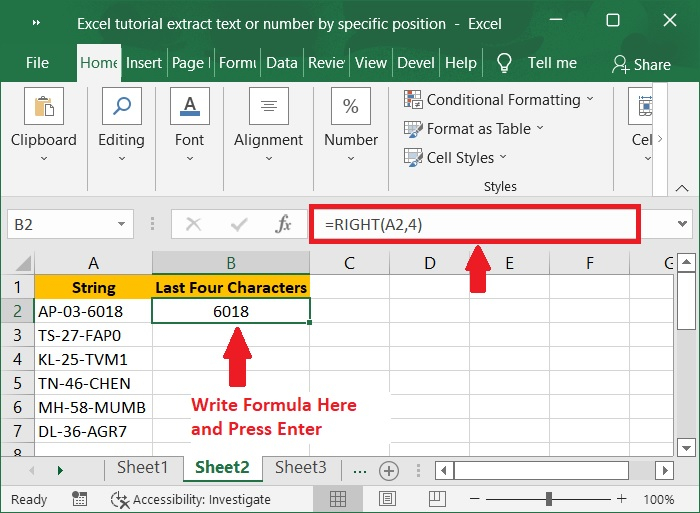Step 3

To apply the formula to the other cells, select the cell that contains the result, then drag the AutoFill Handle down. Below is the screenshot for the same.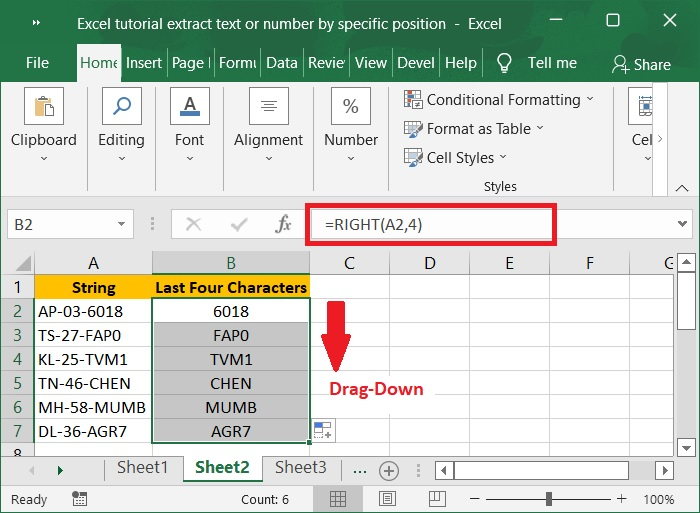## Extract text before the first delimiter

Step 1

To retrieve text from a cell that is located before the first delimiter, you can use a formula that is based on the LEFT and FIND functions to assist you. In the first step create the sample data as shown in the below screenshot.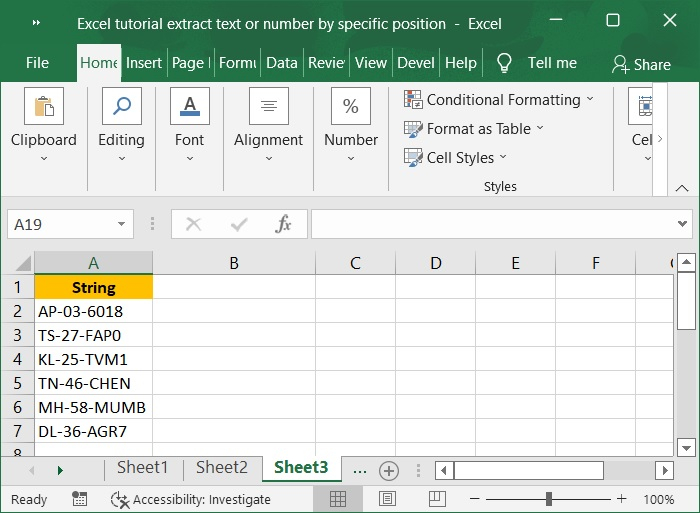Step 2

To extract the first two characters before the first delimiter from the above string in Column A, we need to use LEFT () function along with FIND() function.

For example, if we are trying to extract first two characters before the first delimiter from the row A2, then write â€ś=LEFT(A2,FIND("-",A2,1)- 1)â€ť in cell B2 and press enter. Refer to below screenshot for the same.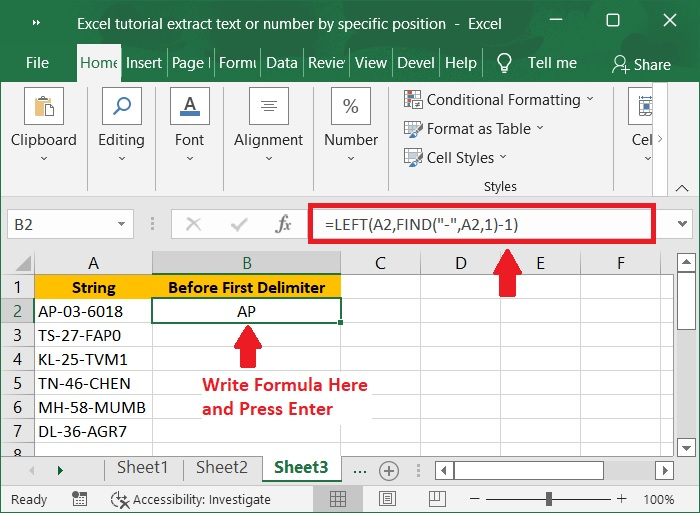Step 3

To apply the formula to the other cells, select the cell that contains the result, then drag the AutoFill Handle down. Below is the screenshot for the same.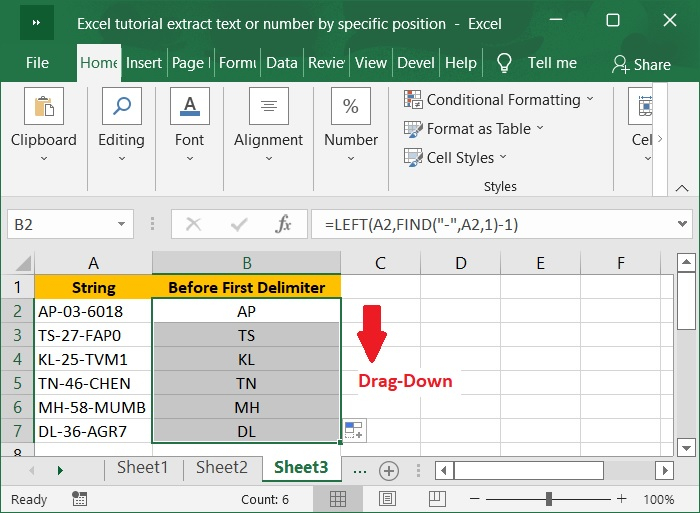## Extract number from the beginning of text string

Step 1

If you are working with data in which text comes after numbers, you can use this generic formula to extract numbers from the beginning of strings so that the text comes before the numbers.

=LEFT(cell,MATCH(FALSE,ISNUMBER(MID(cell,ROW(INDIRECT("1:"&LEN(cell)+1)), 1) *1), 0) -1)


Letâ€™s understand the above formula with a sample data as shown in the below screenshot.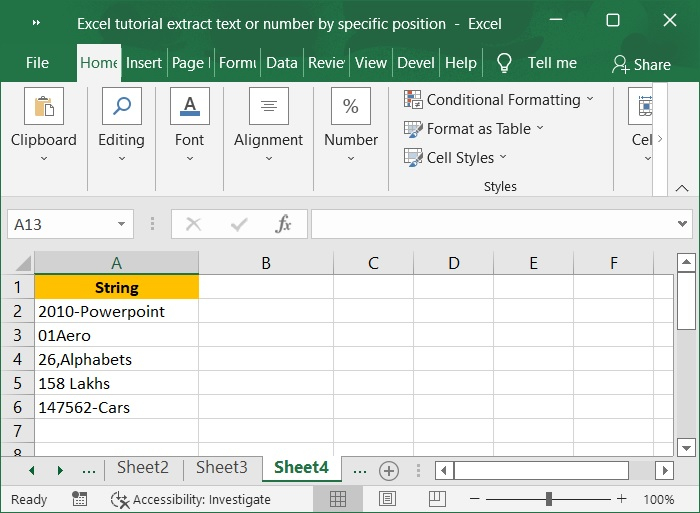Step 2

In the next step, enter the formula in the cell B2 to extract numbers from the beginning of string in cell A1 and press Ctrl+Shift+Enter.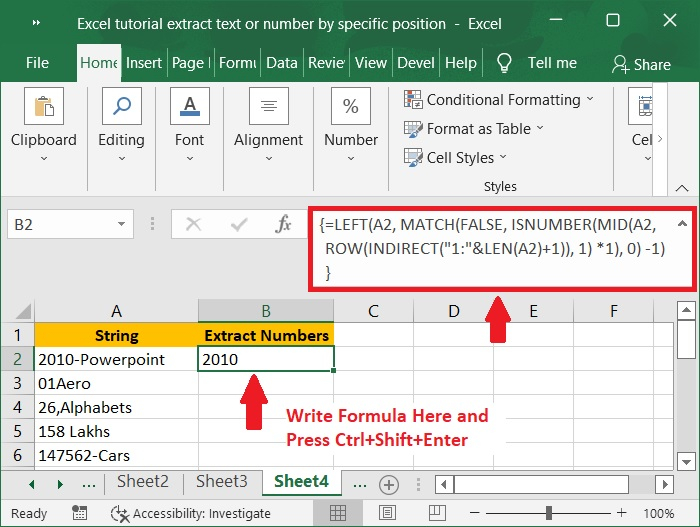Step 3

To apply the formula to the other cells, select the cell that contains the result, then drag the AutoFill Handle down. Below is the screenshot for the same.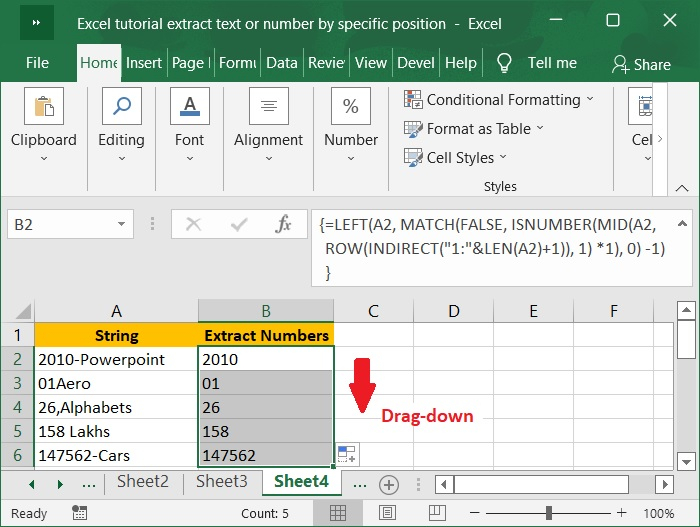## Extract number from the end of text string

Step 1

If you have a column of alphanumeric strings where the number comes after the text, you can use the formula below to get it.

RIGHT(cell,LEN(cell)-MAX(IF(ISNUMBER(MID(cell,ROW(INDIRECT("1:"&LEN(cell))),1)*1)=FALSE, ROW(INDIRECT("1:"&LEN(cell))), 0)))


Letâ€™s understand the above formula with a sample data as shown in the below screenshot.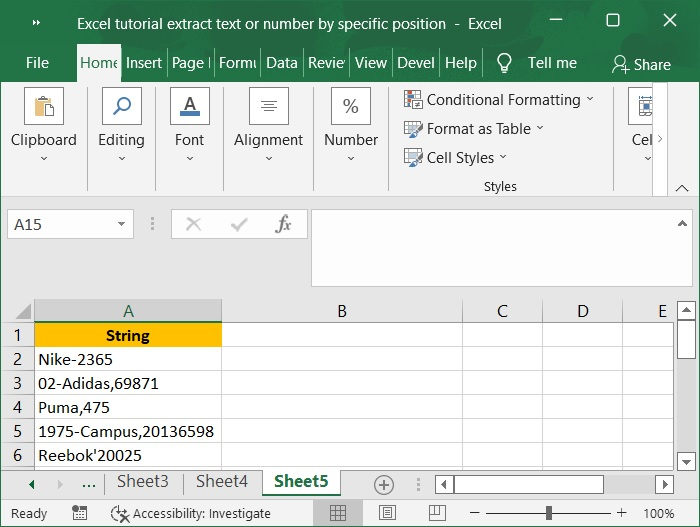Step 2

In the next step, enter the formula in the cell B2 to extract numbers from the end of string from cell A1 and press Ctrl+Shift+Enter.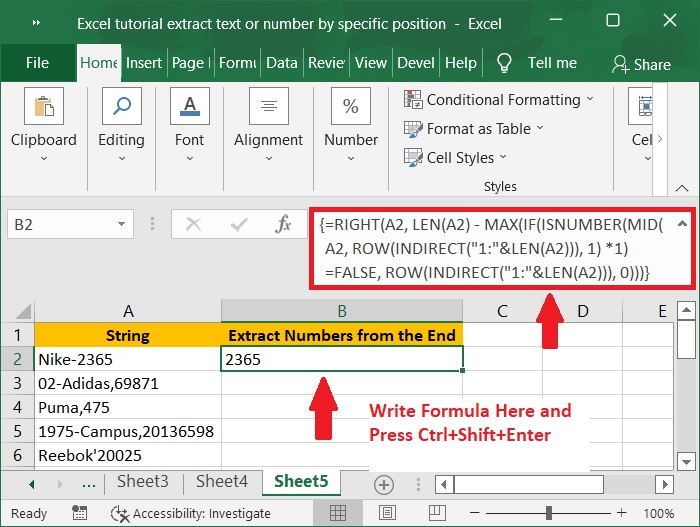Step 3

To apply the formula to the other cells, select the cell that contains the result, then drag the AutoFill Handle down. Below is the screenshot for the same.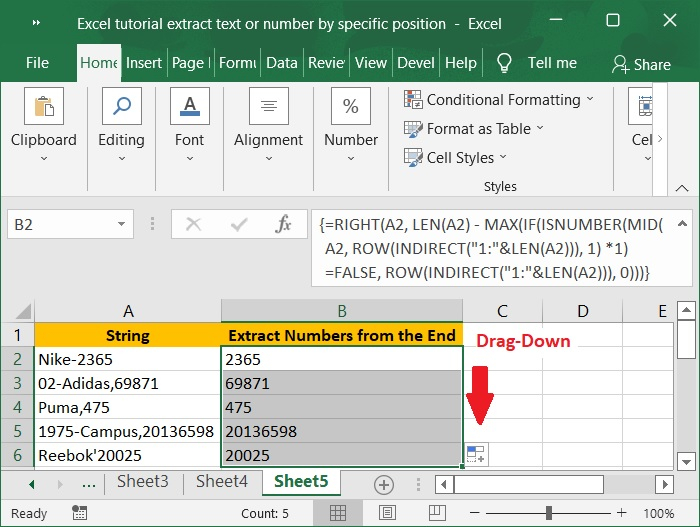## Conclusion

In many circumstances, you may merely need to extract valuable content from a sentence or text string in a cell, such as extracting the province from an address or extracting the email address from a sentence. This article helps us to understand the steps to extract text or number from specific positions.